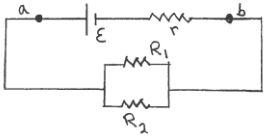Problem: The circuit shown in the sketch consists of a battery with emf ε and internal resistance r connected to two resistors as shown. R1 = 3.0 Ω, R2 = 6.0 Ω and r = 0.50 Ω. The terminal voltage Vab of the battery is 14.0 V.  What is the emf of the battery?

FREE Expert Solution
87% (366 ratings)
Problem Details

The circuit shown in the sketch consists of a battery with emf ε and internal resistance connected to two resistors as shown. R1 = 3.0 Ω, R2 = 6.0 Ω and r = 0.50 Ω. The terminal voltage Vab of the battery is 14.0 V.  What is the emf of the battery?